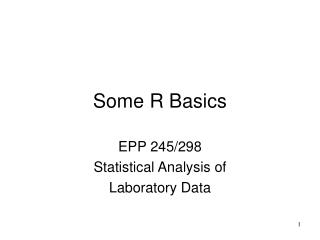DownloadDownload PresentationSome R Basics

# Some R Basics

Download Presentation## Some R Basics

- - - - - - - - - - - - - - - - - - - - - - - - - - - E N D - - - - - - - - - - - - - - - - - - - - - - - - - - -
##### Presentation Transcript

1. Some R Basics EPP 245/298 Statistical Analysis of Laboratory Data

2. Getting Data into R • Many times the most direct method is to edit the data in Excel, Export as a txt file, then import to R using read.delim • We will do this two ways for the energy data from Dalgaard • Frequently, the data from studies I am involved in arrives in Excel EPP 245 Statistical Analysis of Laboratory Data

3. > eg <- read.delim("energy1.txt") > eg Obese Lean 1 9.21 7.53 2 11.51 7.48 3 12.79 8.08 4 11.85 8.09 5 9.97 10.15 6 8.79 8.40 7 9.69 10.88 8 9.68 6.13 9 9.19 7.90 10 NA 7.05 11 NA 7.48 12 NA 7.58 13 NA 8.11 EPP 245 Statistical Analysis of Laboratory Data

4. > class(eg)  "data.frame" > t.test(eg\$Obese,eg\$Lean) Welch Two Sample t-test data: eg\$Obese and eg\$Lean t = 3.8555, df = 15.919, p-value = 0.001411 alternative hypothesis: true difference in means is not equal to 0 95 percent confidence interval: 1.004081 3.459167 sample estimates: mean of x mean of y 10.297778 8.066154 > mean(eg\$Obese)-mean(eg\$Lean)  NA > mean(eg\$Obese[1:9])-mean(eg\$Lean)  2.231624 > EPP 245 Statistical Analysis of Laboratory Data

5. > class(eg)  "data.frame" > t.test(eg\$Obese,eg\$Lean) Welch Two Sample t-test data: eg\$Obese and eg\$Lean t = 3.8555, df = 15.919, p-value = 0.001411 alternative hypothesis: true difference in means is not equal to 0 95 percent confidence interval: 1.004081 3.459167 sample estimates: mean of x mean of y 10.297778 8.066154 > mean(eg\$Obese)-mean(eg\$Lean)  NA > mean(eg\$Obese[1:9])-mean(eg\$Lean)  2.231624 > EPP 245 Statistical Analysis of Laboratory Data

6. > eg2 <- read.delim("energy2.txt") > eg2 expend stature 1 9.21 Obese 2 11.51 Obese 3 12.79 Obese 4 11.85 Obese 5 9.97 Obese 6 8.79 Obese 7 9.69 Obese 8 9.68 Obese 9 9.19 Obese 10 7.53 Lean 11 7.48 Lean 12 8.08 Lean 13 8.09 Lean 14 10.15 Lean 15 8.40 Lean 16 10.88 Lean 17 6.13 Lean 18 7.90 Lean 19 7.05 Lean 20 7.48 Lean 21 7.58 Lean 22 8.11 Lean EPP 245 Statistical Analysis of Laboratory Data

7. > class(eg2)  "data.frame" > t.test(eg2\$expend ~ eg2\$stature) Welch Two Sample t-test data: eg2\$expend by eg2\$stature t = -3.8555, df = 15.919, p-value = 0.001411 alternative hypothesis: true difference in means is not equal to 0 95 percent confidence interval: -3.459167 -1.004081 sample estimates: mean in group Lean mean in group Obese 8.066154 10.297778 > mean(eg2[eg2[,2]=="Lean",1])-mean(eg2[eg2[,2]=="Obese",1])  -2.231624 EPP 245 Statistical Analysis of Laboratory Data

8. > mean(eg2[eg2[,2]=="Lean",1])-mean(eg2[eg2[,2]=="Obese",1])  -2.231624 > tapply(eg2[,1],eg2[,2],mean) Lean Obese 8.066154 10.297778 > tmp <-tapply(eg2[,1],eg2[,2],mean) > tmp Lean Obese 8.066154 10.297778 > class(tmp)  "array" > dim(tmp)  2 > tmp-tmp Lean -2.231624 EPP 245 Statistical Analysis of Laboratory Data